TORSCHE Scheduling Toolbox for Matlab

TORSCHE – Scheduling Algorithms

Scheduling algorithms are the most interesting part of the toolbox. This section deal with scheduling on monoprocessor/dedicated processors/parallel processors and with cyclic scheduling. The scheduling algorithms are categorized by notation (α | β | γ) proposed by [Graham79] and [Błażewicz83].

1. Structure of Scheduling Algorithms

Scheduling algorithm in TORSCHE is a Matlab function with at least two input parameters and at least one output parameter. The first input parameter must be taskset, with tasks to be scheduled. The second one must be an instance of problem object describing the reguired scheduling problem in (α | β | γ) notation. Taskset containing resulting schedule must be the first output parameter. Common syntax of the scheduling algorithms calling is:

`TS = name(T,problem[,processors[,parameters]])`
name

command name of algorithm

TS

set of tasks with schedule inside

T

set of tasks to be scheduled

problem

object of type problem describing the classification of deterministic scheduling problems

processors

number of processors for which schedule is computed

parameters

additional information for algorithms, e.g. parameters of mathematical solvers etc.

The common structure of scheduling algorithms is depicted in Figure 7.1, “Structure of scheduling algorithms in the toolbox.”. First of all the algorithm must check whether the reguired scheduling problem can be solved by himself. In this case the function `is` is used as is shown in part "scheduling problem check". Further, algorithm should perform initialization of variables like `n` (number of tasks), `p` (vector of processing times), ... Then a scheduling algorithm calculates start time of tasks (`starts`) and processor assignemen (`processor`) - if required. Finaly the resulting schedule is derived from the original taskset using function `add_schedule`.

```function [TS] = schalg(T,problem)
%function description

%scheduling problem check
if ~(is(prob,'alpha','P2') && is(prob,'betha','rj,prec') && ...
is(prob,'gamma','Cmax'))
error('Can not solve this problem.');
end

%initialization of variables
n = count(T);              %number of tasks
p = T.ProcTime             %vector of processing time

%scheduling algorithm
...
starts = ...               %assignemen of resulting start times
processor = ...            %processor assignemen

%output schedule construction
description = 'a scheduling algorithm';
TS = T;

%end of file```

Figure 7.1. Structure of scheduling algorithms in the toolbox.

2. List of Algorithms

Table 7.1 shows reference for all the scheduling algorithms available in the current version of the toolbox. Each algorithm is described by its full name, command name, problem clasification and reference to literature where the problem is described.

algorithmcommandproblemreference
Algorithm for 1|rj|Cmaxalg1rjcmax1|rj|Cmax[Błażewicz01]
Bratley’s Algorithmbratley1|rj,~dj|Cmax[Błażewicz01]
Hodgson's Algorithmalg1sumuj1||ΣUj[Błażewicz01]
Algorithm for P||CmaxalgpcmaxP||Cmax[Błażewicz01]
McNaughton's AlgorithmmcnaughtonruleP|pmtn|Cmax[Błażewicz01]
Algorithm for P|rj,prec,~dj|CmaxalgprjdeadlinepreccmaxP|`rj`,prec,~`dj`|Cmax
Hu's AlgorithmhuP|in-tree,pj=1|Cmax[Błażewicz01]
Brucker's algorithmbrucker76P|in-tree,pj=1|Lmax[Bru76], [Błażewicz01]
Horn's Algorithmhorn1|pmtn,rj|Lmax[Horn74], [Błażewicz01]
List SchedulinglistschP|prec|Cmax[Graham66], [Błażewicz01]
Coffman's and Graham's AlgorithmcoffmangrahamP2|prec,pj=1|Cmax[Błażewicz01]
Scheduling with Positive and Negative Time-LagsspntlSPNTL[Brucker99], [Hanzalek04]
Cyclic scheduling (General)cycschCSCH[Hanen95], [Sucha04]
SAT SchedulingsatschP|prec|Cmax[TORSCHE06]

Table 7.1. List of algorithms

3. Algorithm for Problem 1|rj|Cmax

This algorithm solves 1|`r`j|Cmax scheduling problem. Tha basic idea of the algorithm is to arrange and schedule the tasks in order of nondecreasing release time `r`j. It is equivalent to the First Come First Served rule (FCFS). The algorithm usage is outlined in Figure 7.1 and the correspondin schedule is displayed in Figure 7.2 as a Gantt chart.

`TS = alg1rjcmax(T,problem)`

```>> T = taskset([3 1 10 6 4]);
>> T.ReleaseTime = ([4 5 0 2 3]);
>> p = problem('1|rj|Cmax');
>> TS = alg1rjcmax(T,p);
>> plot(TS);```

Figure 7.2. Scheduling problem 1|`r`j|Cmax solving.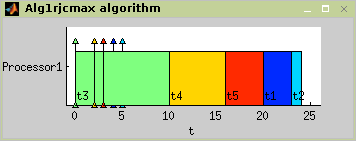Figure 7.3. Alg1rjcmax algorithm - problem 1|`r`j|Cmax

4. Bratley’s Algorithm

Bratley’s algorithm, proposed to solve 1|`r`j,~dj|Cmax problem, is algorithm which uses branch and bound method. Problem is from class NP-hard and finding best solution is based on backtracking in the tree of all solutions. Number of solutions is reduced by testing availabilty of schedule after adding each task. For more details about Bratley’s algorithm see [Błażewicz01].

In Figure 7.3 the algorithm usage is shown. The resulting schedule is shown in Figure 7.4.

`TS = bratley(T,problem)`
```>> T = taskset([2 1 2 2]);
>> T.ReleaseTime = ([4 1 1 0]);
>> T.Deadline = ([7 5 6 4]);
>> p = problem('1|rj,~dj|Cmax');
>> TS = bratley(T,p);
>> plot(TS);```

Figure 7.4. Scheduling problem 1|`r`j,~dj|Cmax solving.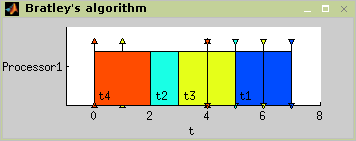Figure 7.5. Bratley’s algorithm - problem 1|`r`j,~dj|Cmax

5. Hodgson's Algorithm

Hodgson's algorithm is proposed to solve 1||ΣUj problem, that means it minimalize number of delayed tasks. Algorithm operates in two steps:

1. The subset Ts of taskset T, that can be processed on time, is determined.

2. A schedule is determined from the subsets Ts and Tn = T – Ts (tasks, that can not be processed on time).

Implementation: Apply EDD (Earliest Due Date First) rule on taskset T. If each task can be processed on time, then this is the final schedule. Else move as much tasks with the longest processing time from Ts to Tn as is needed to process each task from Ts on time. Then schedule subset Tn in an arbitrary order. Final schedule is [Ts Tn]. For more details about Hodgson's algorithm see [Błażewicz01].

In Figure 7.5 the algorithm usage is outlined. The resulting schedule is displayed in Figure 7.6.

`TS = alg1sumuj(T,problem)`
```>> T = taskset([7 8 4 6 6]);
>> T.DueDate = ([9 17 18 19 21]);
>> p = problem('1||sumUj');
>> TS = alg1sumuj(T,p);
>> plot(TS);```

Figure 7.6. Scheduling problem 1||ΣUj solving.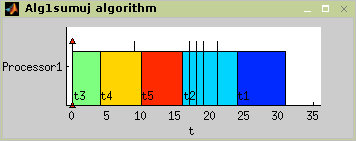Figure 7.7. Hodgson’s algorithm - problem 1||ΣUj

6. Algorithm for Problem P||Cmax

This algorithm solves problem P||Cmax, where a set of independent tasks has to be assigned to parallel identical processors in order to minimize schedule length. Preemption is not allowed. Algorithm finds optimal schedule using Integer Linear Programming (ILP). The algorithm usage is outlined in Figure 7.7 and resulting schedule is displayed in Figure 7.8.

`TS = algpcmax(T,problem,processors)`
```>> T=taskset([7 7 6 6 5 5 4 4 4]);
>> T.Name={'t1' 't2' 't3' 't4' 't5' 't6' 't7' 't8' 't9'};
>> p = problem('P||Cmax');
>> TS = algpcmax(T,p,4);
>> plot(TS);```

Figure 7.8. Scheduling problem P||Cmax solving.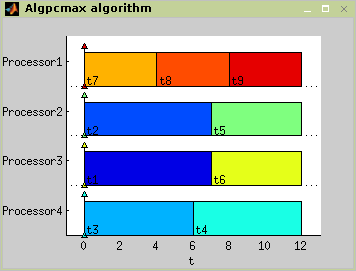Figure 7.9. Algpcmax algorithm - problem P||Cmax

7. McNaughton's Algorithm

McNaughton’s algorithm solves problem P|pmtn|Cmax, where a set of independent tasks has to be scheduled on identical processors in order to minimize schedule length. This algorithm consider preemption of the task and the resulting schedule is optimal. The maximum length of task schedule can be defined as maximum of this two values: max(`p`j); (∑`p`j)/m, where m means number of processors. For more details about Hodgson’s algorithm see [Błażewicz01].

The algorithm use is outlined in Figure 7.9. The resulting Gantt chart is shown in Figure 7.10.

`TS = mcnaughtonrule(T,problem,processors)`

```>> T = taskset([11 23 9 4 9 33 12 22 25 20]);
>> T.Name = {'t1' 't2' 't3' 't4' 't5' 't6' 't7' 't8' 't9' 't10' };
>> p = problem('P|pmtn|Cmax');
>> TS = mcnaughtonrule(T,p,4);
>> plot(TS);```

Figure 7.10. Scheduling problem P|pmtn|Cmax solving.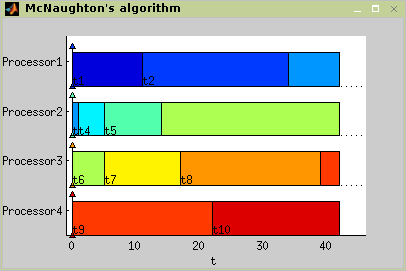Figure 7.11. McNaughton's algorithm - problem P|pmtn|Cmax

8. Algorithm for Problem P|rj,prec,~dj|Cmax

This algorithm is designed for solving P|`r`j,prec,~`d`j|Cmax problem. The algorithm uses modified List Scheduling algorithm List Scheduling to determine an upper bound of the criterion Cmax. The optimal schedule is found using ILP(integer linear programming).

In Figure 7.11 the algorithm usage is shown. The resulting Gantt chart is displayed in Figure 7.12.

`TS = algprjdeadlinepreccmax(T,problem,processors)`
```>> t1 = task('t1',4,0,4);
>> prec = [0 0 0 0 0;...
0 0 0 0 0;...
0 0 0 1 0;...
0 0 0 0 0;...
0 1 0 0 0];
>> T = taskset([t1 t2 t3 t4 t5],prec);
>> prob = problem('P|rj,prec,~dj|Cmax');
>> plot(TS);```

Figure 7.12. Scheduling problem P|`p`j,prec,~`d`j|Cmax solving.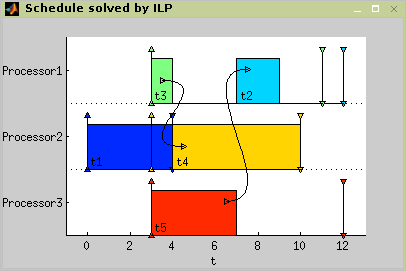Figure 7.13. Algprjdeadlinepreccmax algorithm - problem P|`p`j,prec,~`d`j|Cmax

9. List Scheduling

List Scheduling (LS) is a heuristic algorithm in which tasks are taken from a pre-specified list. Whenever a machine becomes idle, the first available task on the list is scheduled and consequently removed from the list. The availability of a task means that the task has been released. If there are precedence constraints, all its predecessors have already been processed. [Leung04] The algorithm terminates when all the tasks from the list are scheduled. In multiprocessor case, the processor with minimal actual time is taken in each iteration of the algorithm.

Heuristic (suboptimal) algorithms do not guarantee finding the optimal. A subset of heuristic algorithms constitute approximation algorithms . It is a group of heuristic algorithms with analytically evaluated accuracy. The accuracy is measured by absolute performance ratio. For example when the objective of scheduling is to minimize `C`max , absolute performance ratio is defined as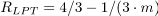, where `C`max`(A(I))` is `C`maxobtained by approximation algorithm `A`, `C`max(OPT(I)) is `C`maxobtained by an optimal algorithm [Błażewicz01] and Π is a set of all instances of the given scheduling problem. For an arbitrary List Scheduling algorithm is proved that `R`LS`=2-1/m`, where `m` is the number of processors. Time complexity of the LS algorithm is `O(n)`.

List Scheduling algorithm is implemented in Scheduling Toolbox as function:

`TS = listsch(T,problem,processors [,strategy])`
`TS = listsch(T,problem,processors [,schoptions])`
T

problem

object problem

processors

number of processors

strategy

strategy for LS algorithm

schoptions

optimization options (see Section Scheduling Toolbox Options)

The algorithm is able to solve R|prec|Cmax or any easier problem. For more details about List Scheduling algorithm see [Błażewicz01].

The set of tasks contains five tasks named {`t1', `t2', `t3', `t4', `t5'} with processing times [2 3 1 2 4]. The tasks are constrained by precedence constraints as shown in Figure 7.14, “An example of P|prec|Cmax scheduling problem.”.

Example 7.1. List Scheduling - problem P|prec|Cmax.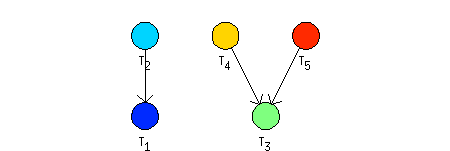Figure 7.14. An example of P|prec|Cmax scheduling problem.

```>> t1=task('t1',2);

>> prec = [0 0 0 0 0;...
1 0 0 0 0;...
0 0 0 0 0;...
0 0 1 0 0;...
0 0 1 0 0];

>> T = taskset([t1 t2 t3 t4 t5],prec);
>> p = problem('P|prec|Cmax');
>> TS = listsch(T,p,2);
>> plot(TS);```

Figure 7.15. Scheduling problem P|prec|Cmax solving.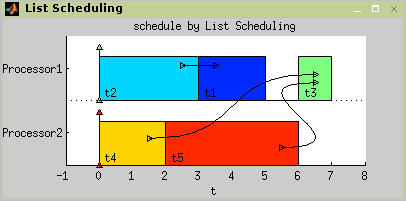Figure 7.16. Result of List Scheduling.

The solution of the example is shown in Figure 7.16, “Result of List Scheduling.”. The LS algorithm found a schedule with `C`max`= 7`.

9.1. LPT

Longest Processing Time first (LPT), intended to solve P||Cmax problem, is a strategy for LS algorithm in which the tasks are arranged in order of non increasing processing time `p`j before the application of List Scheduling algorithm. The time complexity of LPT is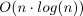. The absolute performance ratio of LPT for problem P||Cmax is[]

LPT is implemented as optional parameter of List Scheduling algorithm and it is able to solve R|prec|Cmax or any easier problem.

`RS = listsch(T,problem,processors,'LPT')`

LS algorithm with LPT strategy demonstrated on the example from previous paragraph is shown in Figure 7.17, “Problem P|prec|Cmax by LS algorithm with LPT strategy solving.”. The resulting schedule with `C`max`= 7` is in Figure 7.18, “Result of LS algorithm with LPT strategy.”.

```>> t1=task('t1',2);

>> prec = [0 0 0 0 0;...
1 0 0 0 0;...
0 0 0 0 0;...
0 0 1 0 0;...
0 0 1 0 0];

>> T = taskset([t1 t2 t3 t4 t5],prec);
>> p = problem('P|prec|Cmax');
>> TS = listsch(T,p,2,'LPT');
>> plot(TS);```

Figure 7.17. Problem P|prec|Cmax by LS algorithm with LPT strategy solving.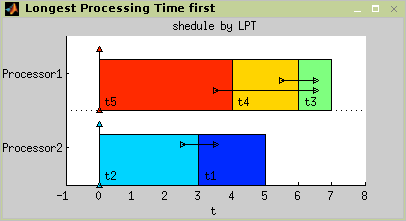Figure 7.18. Result of LS algorithm with LPT strategy.

9.2. SPT

Shortest Processing Time first (SPT), intended to solve P||Cmax problem, is a strategy for LS algorithm in which the tasks are arranged in order of nondecreasing processing time `p`j before the application of List Scheduling algorithm. The time complexity of SPT is also[]

SPT is implemented as optional parameter of List Scheduling algorithm and it is able to solve R|prec|Cmax or any easier problem .

`TS = listsch(T,problem,processors,'SPT')`

LS algorithm with SPT strategy demonstrated on the example from Figure 7.14, “An example of P|prec|Cmax scheduling problem.” is shown in Figure 7.19, “Solving P|prec|Cmax by LS algorithm with SPT strategy.”. The resulting schedule with `C`max`= 7` is in Figure 7.20, “Result of LS algorithm with SPT strategy.”.

```>> t1=task('t1',2);

>> prec = [0 0 0 0 0;...
1 0 0 0 0;...
0 0 0 0 0;...
0 0 1 0 0;...
0 0 1 0 0];

>> T = taskset([t1 t2 t3 t4 t5],prec);
>> p = problem('P|prec|Cmax');
>> TS = listsch(T,p,2,'SPT');
>> plot(TS);```

Figure 7.19. Solving P|prec|Cmax by LS algorithm with SPT strategy.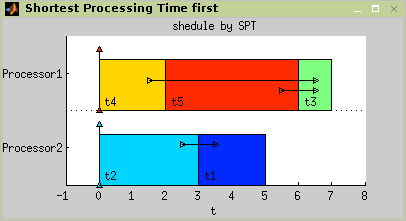Figure 7.20. Result of LS algorithm with SPT strategy.

9.3. ECT

Earliest Completion Time first (ECT), intended to solve P||∑Cj problem, is a strategy for LS algorithm in which the tasks are arranged in order of nondecreasing completion time `C`j in each iteration of List Scheduling algorithm. The time complexity of ECT is equal or better than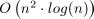.

ECT is implemented as optional parameter of List Scheduling algorithm and it is able to solve R|rj, prec|∑wjCj or any easier problem.

`TS = listsch(T,problem,processors,'ECT')`

An example of P|rj|∑wjCj scheduling problem given with set of five tasks with names, processing time and release time is shown in Table 7.2, “An example of P|rj|∑wjCj scheduling problem.”. The schedule obtained by ECT strategy with ∑`C`j = 58 is shown in Figure 7.24, “Result of LS algorithm with EST strategy.”.

 name processing time release time t1 3 10 t2 5 9 t3 5 7 t4 5 2 t5 9 0

Table 7.2. An example of P|rj|∑wjCj scheduling problem.

```>> t1=task('t1',3,10);

>> T = taskset([t1 t2 t3 t4 t5]);

>> p = problem('P|rj|sumCj');
>> TS = listsch(T,p,2,'ECT');
>> plot(TS);```

Figure 7.21. Solving P|rj|∑Cj by ECT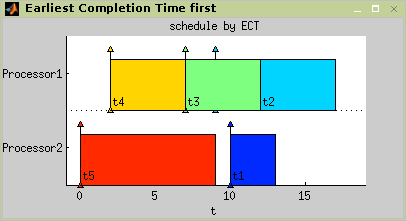Figure 7.22. Result of LS algorithm with ECT strategy.

9.4. EST

Earliest Starting Time first (EST), intended to solve P||∑Cj problem, is a strategy for LS algorithm in which the tasks are arranged in order of nondecreasing starting time `r`j before the application of List Scheduling algorithm. The time complexity of EST is.

EST is implemented as an optional parameter to List Scheduling algorithm and it is able to solve R|rj, prec|∑wjCj or any easier problem.

`TS = listsch(T,problem,processors,'EST')`

LS algorithm with EST strategy demonstrated on the example from Figure 7.14, “An example of P|prec|Cmax scheduling problem.” is shown in Figure 7.23, “Problem P|rj|∑Cj by LS algorithm with EST strategy solving.”. The resulting schedule with ∑`C`j = 57 is in Figure 7.24, “Result of LS algorithm with EST strategy.”.

```>> t1=task('t1',3,10);

>> T = taskset([t1 t2 t3 t4 t5]);

>> p = problem('P|rj|sumCj');
>> TS = listsch(T,p,2,'EST');
>> plot(TS);```

Figure 7.23. Problem P|rj|∑Cj by LS algorithm with EST strategy solving.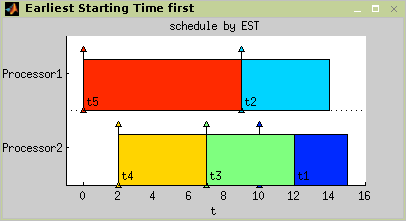Figure 7.24. Result of LS algorithm with EST strategy.

9.5. Own Strategy Algorithm

It's possible to define own strategy for LS algorithm according to the following model of function. Function with the same name as the optional parameter (name of strategy function) is called from List Scheduling algorithm:

`TS = listsch(T,problem,processors,'OwnStrategy')`

In this case, strategy algorithm is called in each iteration of List Scheduling algorithm upon the set of unscheduled task. Strategy algorithm is a standalone function with following parameters:

`[TS, order] = OwnStrategy(T[,iteration,processor]);`
T

order

index vector representing new order of tasks

iteration

actual iteration of List Scheduling algorithm

processor

selected processor

The internal structure of the function can be similar to implementation of EST strategy in private directory of scheduling toolbox.

```function [TS, order] = OwnStrategy(T, varargin) % head

% body
if nargin>1
if varargin{1}>1
return
end
end

[TS order] = sort(T,wreltime,'inc'); % sort taskset
% end of body```

Figure 7.25. An example of OwnStrategy function.

Standard variable `varargin` represents optional parameters `iteration` and `processor`. The definition of this variable is required in the head of function when it is used with `listsch`.

10. Brucker’s Algorithm

Brucker’s algorithm, proposed to solve 1|`in-tree,p`j=1|Lmax problem, is an algorithm which can be implemented in O(n.log n) time [Bru76][]. Implementation in the toolbox use listscheduling algorithm while tasks are sorted in non-increasing order of theyr modified due dates subject to precedence constraints. The algorithm returns an optimal schedule with respect to criterion Lmax. Parameters of the function solving this scheduling problem are described in the Reference Guide brucker76.m.

Examples in Figure 7.26, “Scheduling problem 1|`in-tree,p`j=1|Lmax solving.” and Figure 7.27, “Brucker’s algorithm - problem 1|`in-tree,p`j=1|Lmax” show, how an instance of the scheduling problem [Błażewicz01] can be solved by the Brucker's algorithm. For more details see `brucker76_demo` in `\scheduling\stdemos`.

```>> load brucker76_demo
There are precedence constraints
>> prob = problem('P|in-tree,pj=1|Lmax');
>> TS = brucker76(T,prob,4);
>> plot(TS);```

Figure 7.26. Scheduling problem 1|`in-tree,p`j=1|Lmax solving.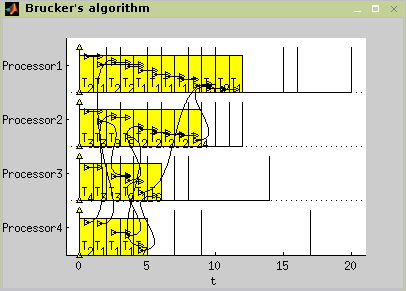Figure 7.27. Brucker’s algorithm - problem 1|`in-tree,p`j=1|Lmax

11. Scheduling with Positive and Negative Time-Lags

Traditional scheduling algorithms (e.g., Błażewicz01) typically assume that deadlines are absolute. However in many real applications release date and deadline of tasks are related to the start time of another tasks [Brucker99][Hanzalek04]. This problem is in literature called scheduling with positive and negative time-lags.

The scheduling problem is given by a task-on-node graph `G`. Each task `t`i is represented by node `t`i in graph G and has a positive processing time `p`i. Timing constraints between two nodes are represented by a set of directed edges. Each edge `e`ij from the node `t`i to the node `t`j is labeled with an integer time lag `w`ij. There are two kinds of edges: the forward edges with positive time lags and the backward edges with negative time lags. The forward edge from the node ti to the node `t`j with the positive time lag `w`ij indicates that `s`j, the start time of `t`j, must be at least `w`ij time units after `s`i, the start time of `t`i. The backward edge from node `t`j to node ti with the negative time lag `w`ji indicates that `s`j must be no more than `w`ji time units after `s`i. The objective is to find a schedule with minimal `C`max.

Since the scheduling problem is NP-hard [Brucker99], algorithm implemented in the toolbox is based on branch and bound algorithm. Alternative implemented solution uses Integer Linear Programming (ILP). The algorithm call has the following syntax:

`TS = spntl(T,problem,schoptions)`
problem

an object of type problem describing the classification of deterministic scheduling problems (see Section Chapter 5, Classification in Scheduling). In this case the problem with positive and negative time lags is identified by `SPNTL'.

schoptions

optimization options (see Section Scheduling Toolbox Options)

The algorithm can be chosen by the value of parameter `schoptions` - structure `schoptions` (see Scheduling Toolbox Options). For more details on algorithms please see [Hanzalek04].

An example of the scheduling problem containing five tasks is shown in Figure 7.28, “Graph `G` representing tasks constrained by positive and negative time-lags.” by graph `G`. Execution times are `p=(1,3,2,4,5)` and delay between start times of tasks t1 and `t`5 have to be less then or equal to 10 (`w`5,1`=-10`). The objective is to find a schedule with minimal `C`max.

Example 7.2. Example of Scheduling Problem with Positive and Negative Time-Lags.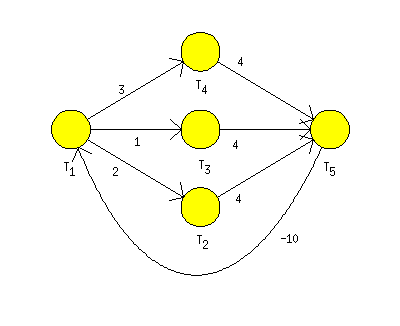Figure 7.28. Graph `G` representing tasks constrained by positive and negative time-lags.

Solution of this scheduling problem using `spntl` function is shown below. Graph of the example can be found in Scheduling Toolbox directory ```<Matlab root>\toolbox\scheduling\stdemos\benchmarks\spntl\spntl_graph.mat```. The graph `G` corresponding to the example shown in Figure 7.28, “Graph `G` representing tasks constrained by positive and negative time-lags.” can be opened and edited in Graphedit tool (`graphedit(g)`).

Resulting graph `G` is shown in Figure 7.31, “Graph `G` weighted by `l`ij and `h`ij of WDF.”. Finaly, the graph `G` is used to generate an object taskset describing the scheduling problem. Parameters conversion must be specified as parameters of function taskset. For example in our case, the function is called with following parameters:

```T = taskset(LHgraph,'n2t',@node2task,'ProcTime','Processor', ...
'e2p',@edges2param)```

For more details see Section 5, “Transformations Between Objects `taskset` and `graph`. The optimal solution in Figure 7.29, “Resulting schedule of instance in Figure 7.28, “Graph `G` representing tasks constrained by positive and negative time-lags.”.” was obtained in the toolbox as is depicted below.

```>> load `<Matlab root>\toolbox`\scheduling\stdemos\benchmarks\spntl_graph
>> graphedit(g)
'e2p',@edges2param)
There are precedence constraints
>> prob=problem('SPNTL')
SPNTL
>> schoptions=schoptionsset('spntlMethod','BaB');
>> T = spntl(T, prob, schoptions)
There are precedence constraints
There is schedule: SPNTL - BaB algorithm
>> plot(t)```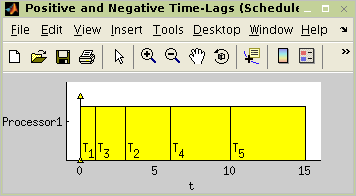Figure 7.29. Resulting schedule of instance in Figure 7.28, “Graph `G` representing tasks constrained by positive and negative time-lags.”.

12. Cyclic Scheduling

Many activities e.g. in automated manufacturing or parallel computing are cyclic operations. It means that tasks are cyclically repeated on machines. One repetition is usually called an iteration and common objective is to find a schedule that maximises throughput. Many scheduling techniques leads to overlapped schedule, where operations belonging to different iterations can execute simultaneously.

Cyclic scheduling deals with a set of operations (generic tasks ti) that have to be performed infinitely often [Hanen95]. Data dependencies of this problem can be modeled by a directed graph `G`. Each task `t`i is represented by the node `t`i in the graph `G` and has a positive processing time `p`i. Edge `e`ij from the node ti to tj is labeled by a couple of integer constants `l`ij and `h`ij. Length `l`ij represents the minimal distance in clock cycles from the start time of the task `t`i to the start time of `t`j and it is always greater than zero. On the other hand, the height `h`ij specifies the shift of the iteration index (dependence distance) from task `t`i to task tj.

Assuming periodic schedule with period `w,` i.e. the constant repetition time of each task, the aim of the cyclic scheduling problem [Hanen95] is to find a periodic schedule with minimal period `w`. In modulo scheduling terminology, period `w` is called Initiation Interval (II).

The algorithm available in this version of the toolbox is based on work presented in [Hanzalek07] and [Sucha07]. Function `cycsch` solves cyclic scheduling of tasks with precedence delays on dedicated sets of parallel identical processors. The algorithm uses Integer Linear Programming

`TS = cycsch(T,problem,m,schoptions)`
problem

object of type problem describing the classification of deterministic scheduling problems (see Section Chapter 5, Classification in Scheduling). In this case the problem is identified by `CSCH'.

m

vector with number of processors in corresponding groups of processors

schoptions

optimization options (see Section Scheduling Toolbox Options)

In addition, the algorithm minimizes the iteration overlap [Sucha04]. This secondary objective of optimization can be disabled in parameter `schoptions`, i.e. parameter `secondaryObjective` of `schoptions` structure (see Scheduling Toolbox Options). The optimization option also allows to choose a method for Cyclic Scheduling algorithm, specify another ILP solver, enable/disable elimination of redundant binary decision variables and specify another ILP solver for elimination of redundant binary decision variables.

For more details on the algorithm please see [Sucha04].

An example of an iterative algorithm used in Digital Signal Processing as a benchmark is Wave Digital Filter (WDF) Fettweis86.

```for k=1 to N do
a(k) =X(k) + e(k-1)  %T1
b(k) = a(k) - g(k-1) %T2
c(k) = b(k) + e(k)   %T3
d(k) = gamma1 * b(k) %T4
e(k) = d(k) + e(k-1) %T5
f(k) = gamma2 * b(k) %T6
g(k) = f(k) + g(k-1) %T7
Y(k) = c(k) - g(k)   %T8
end```

The corresponding Cyclic Data Flow Graph is shown in Figure 7.30, “Cyclic Data Flow Graph of WDF.”. Constant on nodes indicates the number of dedicated group of processors. The objective is to find a cyclic schedule with minimal period `w` on one add and one mul unit. Input-output latency of add (mul) unit is 1 (3) clock cycle(s).

Example 7.3. Cyclic Scheduling - Wave Digital Filter.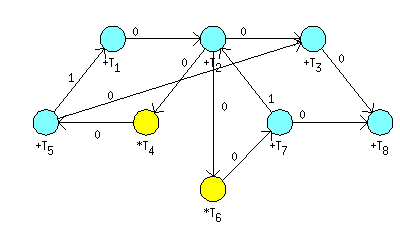Figure 7.30. Cyclic Data Flow Graph of WDF.

To transform Cyclic Data Flow Graph (CDFG) to graph `G` weighted by `l`ij and `h`ij function LHgraph can be used:

`LHgraph = cdfg2LHgraph(dfg,UnitProcTime,UnitLattency)`
LHgraph

graph `G` weighted by `l`ij and `h`ij

dfg

Data Flow Graph where user parameter (UserParam) on nodes represents dedicated processor and user parameter (UserParam) on edges correspond to dependence distance - height of the edge.

UnitProcTime

vector of processing time of tasks on dedicated processors

UnitLattency

vector of input-output latency of dedicated processors

Resulting graph `G` is shown in Figure 7.31, “Graph `G` weighted by `l`ij and `h`ij of WDF.”. Finaly, the graph `G` is used to generate an object taskset describing the scheduling problem. Parameters conversion must be specified as parameters of function taskset. For example in our case, the function is called with following parameters:

```T = taskset(LHgraph,'n2t',@node2task,'ProcTime','Processor', ...
'e2p',@edges2param)```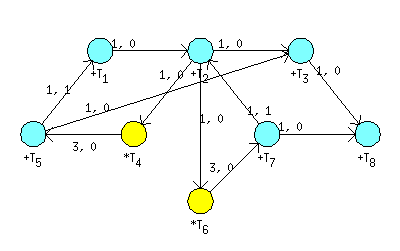Figure 7.31. Graph `G` weighted by `l`ij and `h`ij of WDF.

The scheduling procedure (shown below) found schedule depicted in Figure 7.32, “Resulting schedule with optimal period `w`=8.”.

```>> load `<Matlab root>\toolbox`\scheduling\stdemos\benchmarks\dsp\wdf
>> graphedit(wdf)
>> UnitProcTime = [1 3];
>> UnitLattency = [1 3];
>> m = [1 1];
>> LHgraph = cdfg2LHgraph(wdf,UnitProcTime,UnitLattency)

0     1     0     0     0     0     0     0
0     0     1     0     0     0     1     1
0     0     0     1     0     0     0     0
0     0     0     0     0     0     0     0
0     1     0     1     0     0     0     0
1     0     1     0     0     0     0     0
0     0     0     0     0     1     0     0
0     0     0     0     1     0     0     0
>>
>> graphedit(LHgraph)
'e2p',@edges2param)
There are precedence constraints
>> prob = problem('CSCH');
>> schoptions = schoptionsset('ilpSolver','glpk', ...
'cycSchMethod','integer','varElim',1);
>> TS = cycsch(T, prob, m, schoptions)
There are precedence constraints
There is schedule: General cyclic scheduling algorithm (method:integer)
Solving time: 1.113s
Number of iterations: 4
>> plot(TS,'prec',0)```

Graph of WDF benchmark [Fettweis86] can be found in Scheduling Toolbox directory ```<Matlab root>\toolbox\scheduling\stdemos\benchmarks\dsp\wdf.mat```. Another available benchmarks are DCT [CDFG05], DIFFEQ [Paulin86], IRR [Rabaey91], ELLIPTIC, JAUMANN [Heemstra92], vanDongen [Dongen92] and RLS [Sucha04][Pohl05].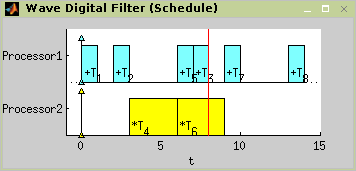Figure 7.32. Resulting schedule with optimal period `w`=8.

13. SAT Scheduling

This section presents the SAT based approach to the scheduling problems. The main idea is to formulate a given scheduling problem in the form of CNF (conjunctive normal form) clauses. TORSCHE includes the SAT based algorithm for P|prec|Cmax problem.

13.1. Instalation

Before use you have to instal SAT solver.

1. Download the zChaff SAT solver (version: 2004.11.15) from the zChaff web site.

2. Place the dowloaded file zchaff.2004.11.15.zip to the `<TORSCHE>\contrib` folder.

3. Be sure that you have C++ compiler set to the mex files compiling. To set C++ compiler call:

`>> mex -setup`

For Windows we tested Microsoft Visual C++ compiler, version 7 and 8. (Version 6 isn't supported.)

For Linux use gcc compiler.

4. From Matlab workspace call m-file `make.m` in `<TORSCHE>\sat` folder.

13.2. Clause preparing theory

In the case of P|prec|Cmax problem, each CNF clause is a function of Boolean variables in the form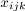. If task ti is started at time unit `j` on the processor `k` then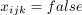, otherwise. For each task `t`i, where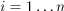, there are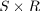Boolean variables, where `S` denotes the maximum number of time units and `R` denotes the total number of processors.

The Boolean variables are constrained by the three following rules (modest adaptation of [Memik02]):

1. For each task, exactly one of thevariables has to be equal to 1. Therefore two clauses are generated for each task ti. The first guarantees having at most one variable equal to 1 (true):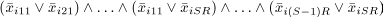The second guarantees having at least one variable equal to 1: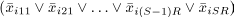2. If there is a precedence constrains such that tu is the predecessor of `t`v, then `t`v cannot start before the execution of `t`u is finished. Therefore,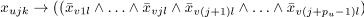for all possible combinations of processors `k` and `l`, where `p`u denotes the processing time of task `t`u.

3. At any time unit, there is at most one task executed on a given processor. For the couple of tasks with a precedence constrain this rule is ensured already by the clauses in the rule number 2. Otherwise the set of clauses is generated for each processor `k` and each time unit `j` for all couples `t`u, `t`v without precedence constrains in the following form: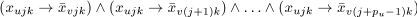In the toolbox we use a zChaff solver to decide whether the set of clauses is satisfiable. If it is, the schedule within `S` time units is feasible. An optimal schedule is found in iterative manner. First, the List Scheduling algorithm is used to find initial value of `S`. Then we iteratively decrement value of `S` by one and test feasibility of the solution. The iterative algorithm finishes when the solution is not feasible.

13.3. Example - Jaumann wave digital filter

As an example we show a computation loop of a Jaumann wave digital filter. Our goal is to minimize computation time of the filter loop, shown as directed acyclic graph in Figure 7.33, “Jaumann wave digital filter”. Nodes in the graph represent the tasks and the edges represent precedence constraints. Green nodes represent addition operations and blue nodes represent multiplication operations. Nodes are labeled by the processing time `p`i. We look for an optimal schedule on two parallel identical processors.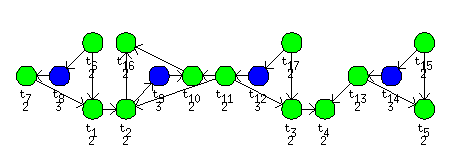Figure 7.33. Jaumann wave digital filter

Folowing code shows consecutive steps performed within the toolbox. First, we define the set of task with precedence constrains and then we run the scheduling algorithm `satsch`. Finally we plot the Gantt chart.

```>> procTime = [2,2,2,2,2,2,2,3,3,2,2,3,2,3,2,2,2];
>> prec = sparse(...
[6,7,1,11,11,17,3,13,13,15,8,6,2,9 ,11,12,17,14,15,2 ,10],...
[1,1,2,2 ,3 ,3 ,4,4 ,5 ,5 ,7,8,9,10,10,11,12,13,14,16,16],...
[1,1,1,1 ,1 ,1 ,1,1 ,1 ,1 ,1,1,1,1 ,1 ,1 ,1 ,1 ,1 ,1 ,1],...
17,17);
>> jaumannSchedule = satsch(jaumann,problem('P|prec|Cmax'),2)
There are precedence constraints
There is schedule: SAT solver
SUM solving time: 0.06s
MAX solving time: 0.04s
Number of iterations: 2
>> plot(jaumannSchedule)```

The `satsch` algorithm performed two iterations. In the first iteration 3633 clauses with 180 variables were solved as satisfiable for `S`=19 time units. In the second iteration 2610 clauses with 146 variables were solved with unsatisfiable result for `S`=18 time units. The optimal schedule is depicted in Figure 7.34, “The optimal schedule of Jaumann filter”.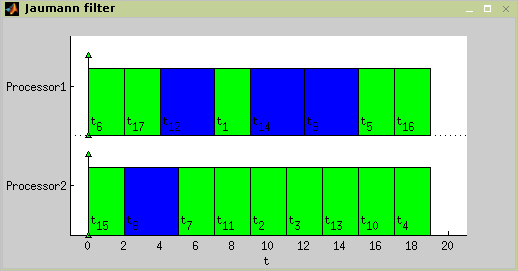Figure 7.34. The optimal schedule of Jaumann filter

14. Hu's Algorithm

Hu's algorithm is intend to schedule unit length tasks with in-tree precedence constraints. Problem notatin is P|in-tree,`p`j=1|Cmax. The algorithm is based on notation of in-tree levels, where in-tree level is number of tasks on path to the root of in-tree graph. The time complexity is O(n).

`TS = hu(T,problem,processors[,verbose])`

or

`TS = hu(T,problem,processors[,schoptions])`
verbose

level of verbosity

schoptions

optimization options

For more details about Hu's algorithm see [Błażewicz01].

There are 12 unit length tasks with precedence constraints defined as in Figure 7.35, “An example of in-tree precedence constraints”.

Example 7.4. Hu's algorithm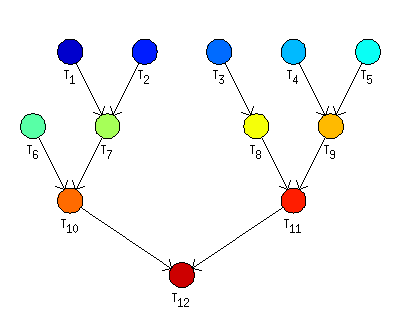Figure 7.35. An example of in-tree precedence constraints

```>> t1=task('t1',1);

>> p = problem('P|in-tree,pj=1|Cmax');
>> prec = [
0 0 0 0 0 0 1 0 0 0 0 0
0 0 0 0 0 0 1 0 0 0 0 0
0 0 0 0 0 0 0 1 0 0 0 0
0 0 0 0 0 0 0 0 1 0 0 0
0 0 0 0 0 0 0 0 1 0 0 0
0 0 0 0 0 0 0 0 0 1 0 0
0 0 0 0 0 0 0 0 0 1 0 0
0 0 0 0 0 0 0 0 0 0 1 0
0 0 0 0 0 0 0 0 0 0 1 0
0 0 0 0 0 0 0 0 0 0 0 1
0 0 0 0 0 0 0 0 0 0 0 1
0 0 0 0 0 0 0 0 0 0 0 0
];
>> T = taskset([t1 t2 t3 t4 t5 t6 t7 t8 t9 t10 t11 t12],prec);

>> TS= hu(T,p,3);
>> plot(TS); ```

Figure 7.36. Scheduling problem P|in-tree,`p`j=1|Cmax using `hu` command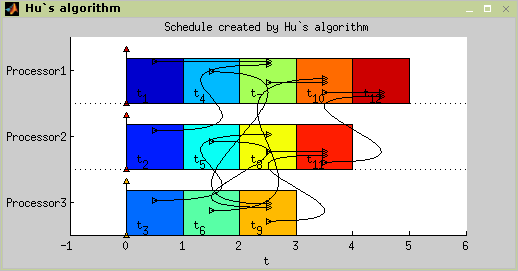Figure 7.37. Hu's algorithm example solution

. Coffman's and Graham's Algorithm

This algorithm generate optimal solution for P2|prec,`p`j=1|Cmax problem. Unit length tasks are scheduled nonpreemptively on two processors with time complexity O(`n`2). Each task is assigned by label, which take into account the levels and the numbers of its imediate successors. Algorithm operates in two steps:

2. Schedule by Hu's algorithm, use labels instead of levels.

`TS = coffmangraham(T,problem[,verbose])`

or

`TS = coffmangraham(T,problem[,schoptions])`
schoptions

optimization options

More about Coffman and Graham algorithm in [Błażewicz01].

The set of tasks contains 13 tasks constrained by precedence constraints as shown in Figure 7.38, “Coffman and Graham example setting”.

Example 7.5. Coffman and Graham algorithm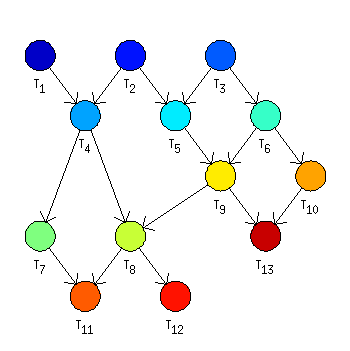Figure 7.38. Coffman and Graham example setting

```>> t1 = task('t1',1);

>> p = problem('P2|prec,pj=1|Cmax');
>> prec = [
0 0 0 1 0 0 0 0 0 0 0 0 0
0 0 0 1 1 0 0 0 0 0 0 0 0
0 0 0 0 1 1 0 0 0 0 0 0 0
0 0 0 0 0 0 1 1 0 0 0 0 0
0 0 0 0 0 0 0 0 1 0 0 0 0
0 0 0 0 0 0 0 0 1 1 0 0 0
0 0 0 0 0 0 0 0 0 0 1 0 0
0 0 0 0 0 0 0 0 0 0 1 1 0
0 0 0 0 0 0 0 1 0 0 0 0 1
0 0 0 0 0 0 0 0 0 0 0 0 1
0 0 0 0 0 0 0 0 0 0 0 0 0
0 0 0 0 0 0 0 0 0 0 0 0 0
0 0 0 0 0 0 0 0 0 0 0 0 0
];
>> T = taskset([t1 t2 t3 t4 t5 t6 t7 t8 t9 t10 t11 t12 t13 t14],prec);

>> TS= coffmangraham(T,p);
>> plot(TS);```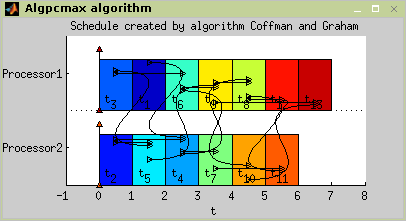Figure 7.39. Coffman and Graham algorithm example solution

Webmaster - Jan Dvořák (2004 - 2019)